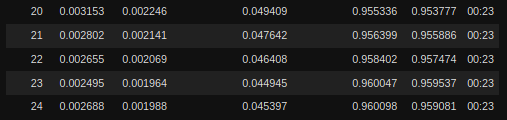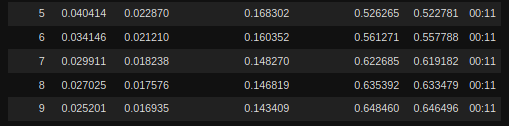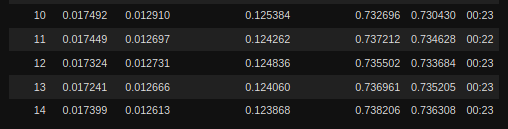# Summary

After having taken the course, one of the core princples taught was transfer learning and how freezing, unfreezing and lr_find can result in great performance by first training only the new layers, then unfreezing and using discrimantive learning rates to train the body at a slower rate than then new head.

1. Freeze pretrained body layers
2. Train for n epochs
3. Unfreeze
4. lr_find
5. train with slice(pretrainedLayerLR, newLayerLR)

# Problem

I’ve setup a regression project that uses two pretrained resnets to review two images, concat the intermediate results and produce an x, y value. During the training process however, I am seeing that the above rules seem to break down completely. In particular, I am getting better results by not freezing at all and just running at 3e-3 than by using lr_find, etc.

# Network

My network code looks like the following:

``````#init weights with kaimingnormal: https://github.com/fastai/fastai/blob/master/fastai/vision/models/xresnet.py#L16
def init_weights(m):
if getattr(m, 'bias', None) is not None: nn.init.constant_(m.bias, 0)
if isinstance(m, (nn.Conv2d, nn.Linear)): nn.init.kaiming_normal_(m.weight)
for l in m.children(): init_weights(l)

class Branch(nn.Module):
def __init__(self):
super(Branch, self).__init__()
self.body = create_body(models.resnet18)

def forward(self, x):
x = self.body(x)
return x

def __init__(self):
nn.BatchNorm1d(1024, eps=1e-05, momentum=0.1, affine=True, track_running_stats=True),
nn.Dropout(p=0.5, inplace=False),
nn.Linear(in_features=1024, out_features=512, bias=True),
nn.LeakyReLU(inplace=True),

nn.BatchNorm1d(512, eps=1e-05, momentum=0.1, affine=True, track_running_stats=True),
nn.Dropout(p=0.5, inplace=False),
nn.Linear(in_features=512, out_features=2, bias=True),
nn.Sigmoid())

def forward(self, x, y):
z = torch.cat([x, y], dim=1)
return z

class YNet(nn.Module):
def __init__(self):
super(YNet, self).__init__()
self.left = Branch()
self.right = Branch()

@staticmethod
def split_layers(m):
groups = [[m.left.body, m.right.body]]
return groups

def forward(self, x, y):
x = self.left(x)
y = self.right(y)
return z
``````

# Training

## Data

``````df = pd.read_csv("recordings/labels.csv")
transforms = get_transforms(do_flip = False, max_rotate = 0, max_zoom = 0, max_warp = 0) #domain specific choices
data = (ImageTupleList.from_df(df, cols=["calibrationPath", "samplePath"], path = ".")
.split_by_rand_pct(0.25, 42)
.label_from_df(cols=[3, 4], label_cls=FloatList)
.transform(transforms)
.databunch(bs=40))
``````

## Without Freezing

``````network = YNet()
learn = Learner(data, network, metrics=[mean_absolute_error, explained_variance, r2_score], loss_func=nn.SmoothL1Loss(), wd=0.1)
learn.split(network.split_layers)

learn.fit_one_cycle(25)
``````## With Freezing

``````network = YNet()
learn = Learner(data, network, metrics=[mean_absolute_error, explained_variance, r2_score], loss_func=nn.SmoothL1Loss(), wd=0.1)
learn.split(network.split_layers)

learn.freeze()
learn.fit_one_cycle(10)
````````````learn.unfreeze()
learn.lr_find()
````````````learn.fit_one_cycle(15, slice(1e-6, 1e-4))
``````# Question

What wrong here? Are there areas where the transfer learning rules of freeze, train, unfreeze, lr_find, train break down? Is my network code bad? Or is the training process broken? Any help to get moving in the right direction would be appreciated.